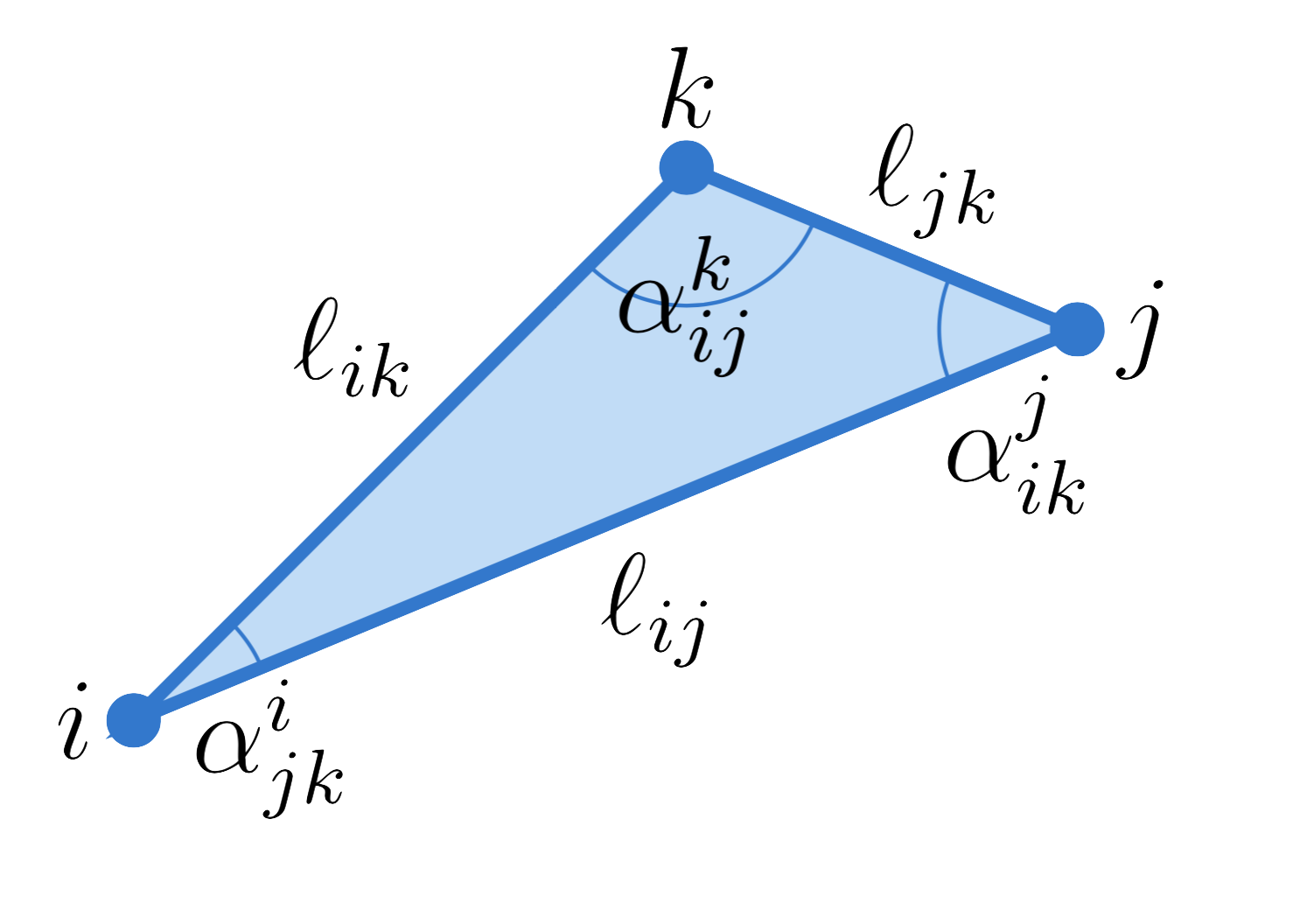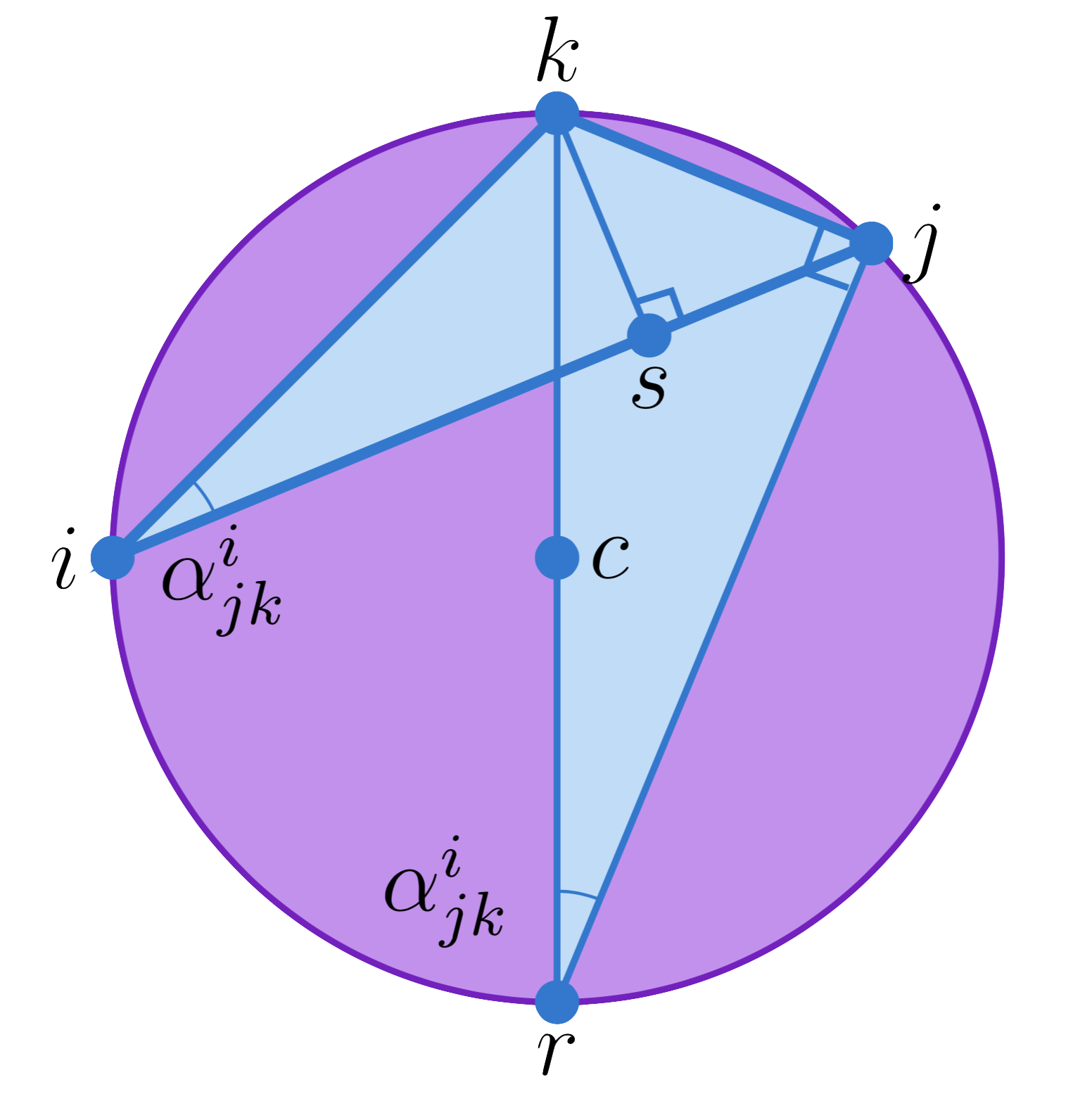## Milnor's Lobachevsky Function ($\text{Л}$)

$\text{Л}(x) := -\int_0^x \log \left| 2 \sin t\right|\;dt$

Note that \begin{aligned} \text{Л}'(x) &= - \log |2 \sin x| \\ \text{Л}''(x) &= -\cot x \end{aligned}

The definition looks very strange. It's probably easiest to think of Л as being defined by the differential equation $\text{Л}''(x) = -\cot x$ subject to the initial conditions \begin{aligned} \text{Л}(0) &= 0\\ \text{Л}'\left(\frac \pi 2\right) &= 0\\ \end{aligned}

## Useful Facts

We begin with a seemingly-unrelated formula about the circumcircle of a triangle.

Let $t_{ijk}$ be a triangle with edge lengths $\ell_{ij}, \ell_{jk}, \ell_{ki}$ and angles $\alpha_{ij}^k, \alpha_{jk}^i, \alpha_{ki}^j$.The radius of the circumcircle of $t_{ijk}$ is given by $R = \frac{\ell_{jk}}{2 \sin \alpha_{jk}^i}$.
Proof

We place our triangle inside of its circumcircle.$c$ is the center of the circle. We draw a diagonal passing though $k$, and name the opposite point $r$. Note that angle $k-j-r$ must be a right angle, since the line $\overline{kr}$ is a diameter of the circle. We also place a point $s$ such that the angle $k-s-j$ is a right angle.

Note that angle $k-r-j$ must equal angle $\alpha_{jk}^i$ since both subtend the same arc between $k$ and $j$. Therefore, triangle $t_{isk}$ is similar to triangle $t_{rjk}$. In particular, this means that $\frac {\ell_{kr}}{\ell_{kj}} = \frac {\ell_{ik}}{\ell_{sk}}$ Note that $\ell_{sk} = \ell_{ik} \sin \alpha_{jk}^i$. Furthermore, $\ell_{rk} = 2R$. Thus, we see that $\frac {2R}{\ell_{kj}} = \frac {\ell_{ik}}{\ell_{ik} \sin \alpha_{jk}^i}$ Simplifying, we conclude that $R = \frac{\ell_{jk}}{2\sin\alpha_{jk}^i}$

### Energy Derivative

Futhermore, let $\lambda_{mn} = 2 \log \ell_{mn}$. We define a function $f( \lambda_{ij}, \lambda_{jk}, \lambda_{ki}) = \frac 12 \big( \alpha_{jk}^i \lambda_{jk} + \alpha_{ki}^j \lambda_{ki} + \alpha_{ij}^k \lambda_{ij}\big) + \text{Л}( \alpha_{jk}^i) + \text{Л}( \alpha_{ki}^j) + \text{Л}( \alpha_{ij}^k)$
$\pd f {\lambda_{jk}} = \frac 12 \alpha_{jk}^i$
Proof

Using the circumradius formula, this becomes a straightforward computation.

\begin{aligned} \pd f {\lambda_{jk}} &= \frac 12 \alpha_{jk}^i + \left(\frac 12\lambda_{jk} - \log |2 \sin \alpha_{jk}^i| \right) \pd {\alpha_{jk}^i}{\lambda_{jk}}\\ &\quad+ \left(\frac 12\lambda_{ki} - \log |2 \sin \alpha_{ki}^j| \right) \pd {\alpha_{ki}^j}{\lambda_{jk}} + \left(\frac 12 \lambda_{ij} - \log |2 \sin \alpha_{ij}^k| \right) \pd {\alpha_{ij}^k}{\lambda_{jk}}\\ &= \frac 12 \alpha_{jk}^i + \log\left(\frac {\ell_{jk}}{2 \sin \alpha_{jk}^i}\right) \pd {\alpha_{jk}^i}{\lambda_{jk}} + \log\left(\frac {\ell_{ki}}{2 \sin \alpha_{ki}^j}\right) \pd {\alpha_{ki}^j}{\lambda_{jk}} + \log\left(\frac {\ell_{ij}}{2 \sin \alpha_{ij}^k}\right) \pd {\alpha_{ij}^k}{\lambda_{jk}} \end{aligned} By the circumradius formula, each of those log terms is just $\log R$. (Equivalently, we could just use the law of sines here to observe that all of the terms are equal. It is not actually important what they are equal to). Thus, we have \begin{aligned} \pd f {\lambda_{jk}} &= \frac 12 \alpha_{jk}^i + \log R \pd{}{\lambda_{jk}} \left(\alpha_{jk}^i + \alpha_{ki}^j + \alpha_{ij}^k\right) \end{aligned} Since the sum of the angles in a triangle is always $\pi$, the derivative on the right vanishes. Thus, we obtain the desired equality \begin{aligned} \pd f {\lambda_{jk}} &= \frac 12 \alpha_{jk}^i \end{aligned}